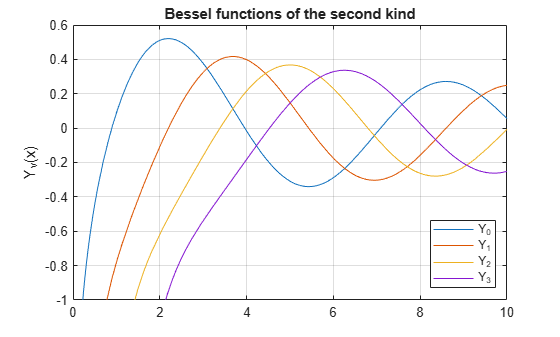# bessely

Bessel function of the second kind for symbolic expressions

## Syntax

``bessely(nu,z)``

## Description

example

````bessely(nu,z)` returns the Bessel function of the second kind, Yν(z).```

## Examples

### Find Bessel Function of Second Kind

Compute the Bessel functions of the second kind for these numbers. Because these numbers are not symbolic objects, you get floating-point results.

`[bessely(0, 5), bessely(-1, 2), bessely(1/3, 7/4), bessely(1, 3/2 + 2*i)]`
```ans = -0.3085 + 0.0000i 0.1070 + 0.0000i 0.2358 + 0.0000i -0.4706 + 1.5873i```

Compute the Bessel functions of the second kind for the numbers converted to symbolic objects. For most symbolic (exact) numbers, `bessely` returns unresolved symbolic calls.

```[bessely(sym(0), 5), bessely(sym(-1), 2),... bessely(1/3, sym(7/4)), bessely(sym(1), 3/2 + 2*i)]```
```ans = [ bessely(0, 5), -bessely(1, 2), bessely(1/3, 7/4), bessely(1, 3/2 + 2i)]```

For symbolic variables and expressions, `bessely` also returns unresolved symbolic calls:

```syms x y [bessely(x, y), bessely(1, x^2), bessely(2, x - y), bessely(x^2, x*y)]```
```ans = [ bessely(x, y), bessely(1, x^2), bessely(2, x - y), bessely(x^2, x*y)]```

### Solve Bessel Differential Equation for Bessel Functions

Solve this second-order differential equation. The solutions are the Bessel functions of the first and the second kind.

```syms nu w(z) dsolve(z^2*diff(w, 2) + z*diff(w) +(z^2 - nu^2)*w == 0)```
```ans = C2*besselj(nu, z) + C3*bessely(nu, z)```

Verify that the Bessel function of the second kind is a valid solution of the Bessel differential equation:

```syms nu z isAlways(z^2*diff(bessely(nu, z), z, 2) + z*diff(bessely(nu, z), z)... + (z^2 - nu^2)*bessely(nu, z) == 0)```
```ans = logical 1```

### Special Values of Bessel Function of Second Kind

If the first parameter is an odd integer multiplied by 1/2, `bessely` rewrites the Bessel functions in terms of elementary functions:

```syms x bessely(1/2, x)```
```ans = -(2^(1/2)*cos(x))/(x^(1/2)*pi^(1/2))```
`bessely(-1/2, x)`
```ans = (2^(1/2)*sin(x))/(x^(1/2)*pi^(1/2))```
`bessely(-3/2, x)`
```ans = (2^(1/2)*(cos(x) - sin(x)/x))/(x^(1/2)*pi^(1/2))```
`bessely(5/2, x)`
```ans = -(2^(1/2)*((3*sin(x))/x + cos(x)*(3/x^2 - 1)))/(x^(1/2)*pi^(1/2))```

### Differentiate Bessel Functions of Second Kind

Differentiate the expressions involving the Bessel functions of the second kind:

```syms x y diff(bessely(1, x)) diff(diff(bessely(0, x^2 + x*y -y^2), x), y)```
```ans = bessely(0, x) - bessely(1, x)/x ans = - bessely(1, x^2 + x*y - y^2) -... (2*x + y)*(bessely(0, x^2 + x*y - y^2)*(x - 2*y) -... (bessely(1, x^2 + x*y - y^2)*(x - 2*y))/(x^2 + x*y - y^2))```

### Find Bessel Function for Matrix Input

Call `bessely` for the matrix `A` and the value 1/2. The result is a matrix of the Bessel functions ```bessely(1/2, A(i,j))```.

```syms x A = [-1, pi; x, 0]; bessely(1/2, A)```
```ans = [ (2^(1/2)*cos(1)*1i)/pi^(1/2), 2^(1/2)/pi] [ -(2^(1/2)*cos(x))/(x^(1/2)*pi^(1/2)), Inf]```

### Plot Bessel Functions of Second Kind

Plot the Bessel functions of the second kind for $v=0,1,2,3$.

```syms x y fplot(bessely(0:3,x)) axis([0 10 -1 0.6]) grid on ylabel('Y_v(x)') legend('Y_0','Y_1','Y_2','Y_3', 'Location','Best') title('Bessel functions of the second kind')```## Input Arguments

collapse all

Input, specified as a number, vector, matrix, or array, or a symbolic number, variable, array, function, or expression.

If `nu` is a vector or matrix, `bessely` returns the Bessel function of the second kind for each element of `nu`.

Input, specified as a number, vector, matrix, or array, or a symbolic number, variable, array, function, or expression.

If `z` is a vector or matrix, `bessely` returns the Bessel function of the second kind for each element of `z`.

collapse all

### Bessel Function of the Second Kind

The Bessel differential equation

`${z}^{2}\frac{{d}^{2}w}{d{z}^{2}}+z\frac{dw}{dz}+\left({z}^{2}-{\nu }^{2}\right)w=0$`

has two linearly independent solutions. These solutions are represented by the Bessel functions of the first kind, Jν(z), and the Bessel functions of the second kind, Yν(z):

`$w\left(z\right)={C}_{1}{J}_{\nu }\left(z\right)+{C}_{2}{Y}_{\nu }\left(z\right)$`

The Bessel functions of the second kind are defined via the Bessel functions of the first kind:

`${Y}_{\nu }\left(z\right)=\frac{{J}_{\nu }\left(z\right)\mathrm{cos}\left(\nu \pi \right)-{J}_{-\nu }\left(z\right)}{\mathrm{sin}\left(\nu \pi \right)}$`

Here Jν(z) are the Bessel function of the first kind:

`${J}_{\nu }\left(z\right)=\frac{{\left(z/2\right)}^{\nu }}{\sqrt{\pi }\Gamma \left(\nu +1/2\right)}\underset{0}{\overset{\pi }{\int }}\mathrm{cos}\left(z\mathrm{cos}\left(t\right)\right)\mathrm{sin}{\left(t\right)}^{2\nu }dt$`

## Tips

• Calling `bessely` for a number that is not a symbolic object invokes the MATLAB® `bessely` function.

At least one input argument must be a scalar or both arguments must be vectors or matrices of the same size. If one input argument is a scalar and the other one is a vector or a matrix, `bessely(nu,z)` expands the scalar into a vector or matrix of the same size as the other argument with all elements equal to that scalar.

 Olver, F. W. J. “Bessel Functions of Integer Order.” Handbook of Mathematical Functions with Formulas, Graphs, and Mathematical Tables. (M. Abramowitz and I. A. Stegun, eds.). New York: Dover, 1972.

 Antosiewicz, H. A. “Bessel Functions of Fractional Order.” Handbook of Mathematical Functions with Formulas, Graphs, and Mathematical Tables. (M. Abramowitz and I. A. Stegun, eds.). New York: Dover, 1972.

#### Mathematical Modeling with Symbolic Math Toolbox

Get examples and videos